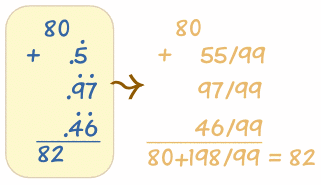# Our Columbus Problem Puzzle - Solution

## The Puzzle:

Here is a famous prize problem that Sam Loyd issued in 1882, offering \$1000 as a prize for the best answer showing how to arrange the seven figures and the eight "dots" .4.5.6.7.8.9.0. which would add up to 82.

Out of several million answers, only two were found to be correct.

## Our Solution:The dot over a number signifies that it is a repeater which would go on for ever, as when we endeavor to describe 1/3 decimally as 0.33333 . . . . (etc)

With a series of numbers we place the dot over the first and last, as with 0.97979797979 . . . (etc)

The remarkable feature being that a proper fraction divided by 9s e.g. 46/99 is exactly equal to the numerator with the repeater sign followed by the decimal.
Puzzle Author: Loyd, Sam

See this puzzle without solution
Discuss this puzzle at the Math is Fun Forum